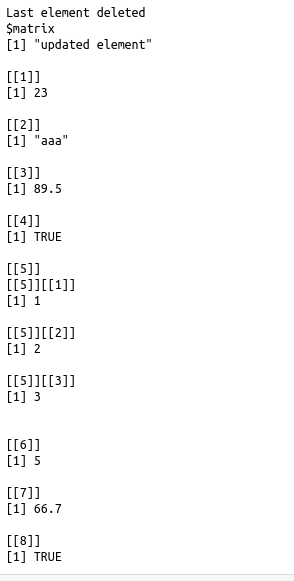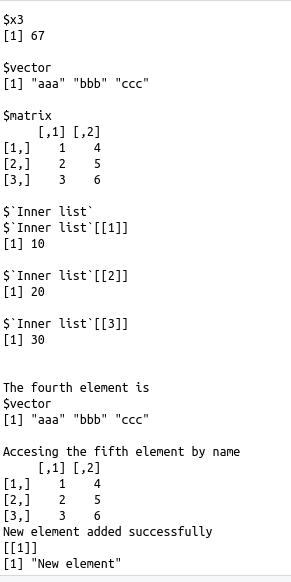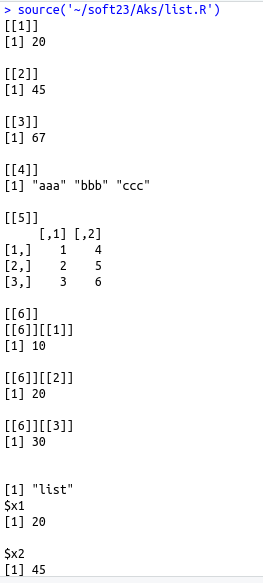• #5, First Floor, 4th Street Dr. Subbarayan Nagar Kodambakkam, Chennai-600 024 Landmark : Samiyar Madam
• pro@slogix.in
• +91- 81240 01111

### What is List in R?

###### Description

To understand the list concept,creating,accesing and manipulating.

###### Process

List creation :

Lists is a collection of elements of different types like numeric,character,matrix,vector,a list and also functions.

Created using function list()

Naming list elements .:

• The elements of the list can be named using the names(listname) function.
• The names are passed are arguments to this function

Syntax :

names(listname)=c(names for the elements)

Accessing list elements :

The elements of the list can be accessed by using their names or by indexing

Manipulating elements :

• Adding an element and deleting an element can be done only at the end of the list.An existing element can be updated.
• Indexing or name can be used to perform this task
• Merging lists :

Merging many into one list can be done by placing all the list inside one list.

Converting list to a vector :

A list can be converted to a vector so that it can be taken to further manipulation.To achieve this the function unlist() is used.

###### Sapmle Code

#Creating a list
a=list(20,45,67,c(“aaa”,”bbb”,”ccc”),matrix(c(1,2,3,4,5,6),nrow=3),list(10,20,30))
print(a)
print(class(a))
#naming the list elements
names(a)<-c(“x1″,”x2″,”x3″,”vector”,”matrix”,”Inner list”)
print(a)
#accessing list elements
cat(“The fourth element is “,”\n”)
print(a)
cat(“Accessing the fifth element by name “,”\n”)
print(a\$matrix)
#manipulating list element
a=”New element”
print(a)
a=NULL
cat(“Last element deleted\n”)
a=”updated element”
print(a)
#merging list
b=list(23,”aaa”,89.5,TRUE,list(1,2,3))
c=list(5,66.7,TRUE,FALSE)
d=c(b,c)
print(d)
print(class(d))
#converting list to a vector
e=unlist(a)
print(class(a))

###### Screenshots